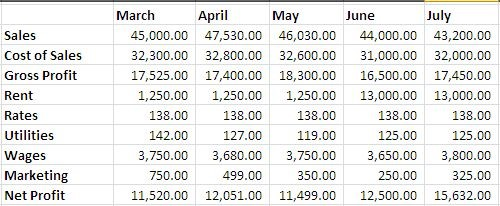# How To VLOOKUP Data For An Entire MonthConsider a case where you have a table like below and want to fetch the data for a single month. What will you do?? The retrieved data should contain values for all the elements in the left pivot column and for a particular month.You can use VLOOKUP() to help you in such a situation. Whereas VLOOKUP is set to look in a particular column, we can make it more dynamic by using MATCH() function. If the header row of the table contains true dates we can use MONTH() function instead to meet the target.

For this case, we will consider that the header row contains true dates, and will try to see how the formula actually works.

The VLOOKUP formula: we have already discussed VLOOKUP in many of our posts. Here is just a quick review of how this formula works.For our case, the lookup value in the left most column and consists of various heads, table array is located in cells B2:B11, The column index needed to be determined dynamically by using MONTH().

So let’s see how this formula is executed.

The formula:

The formula that works here is following.

=VLOOKUP(B16,\$B\$4:\$G\$12,MATCH(MONTH(\$C\$14),MONTH(\$C\$3:\$G\$3),0)+1,0)

Let’s understand this formula by breaking it in parts, let see how it gives us the correct result.

The MATCH() Part:

The match part works by getting the month from the criteria cell C14 and doing the same thing for the range C3:G3, that works as a lookup range. The MATCH function matches it for an exact type of result by using zero as the last argument.

The result is then feed to the VLOOKUP’s third argument that uses it to give the final result.The VLOOKUP() Part:

The VLOOKUP part uses the result of MATCH() function and adds +1 to it to make up for the first column on the left. Then it performs an exact lookup for the given value and when we drag it downwards, ti give us the desired result.You can still produce variations for it – for year or for quarter, it is how well you can use this function.